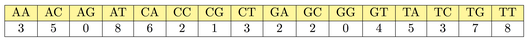# Glossary

## k-mer composition

The $k$-mer composition of a string $s$ encodes the number of times that each possible k-mer occurs in $s$. To represent the k-mer composition of a string concisely, all possible k-mers (in the case of DNA strings, there will be $4^k$ total $k$-mers) are ordered lexicographically, and then an array $A$ is created in which $A[i]$ represents the number of times that the $i$th of these ordered $k$-mers appears in $s$.

The $k$-mer composition is a generalization of GC-content to the case of substrings. In the figure below, we show the array giving the 2-mer composition of "TTGATTACCTTATTTGATCATTACACATTGTACGCTTGTGTCAAAATATCACATGTGCCT".